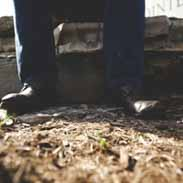# thermochemistry and nuclear chemistry examDaniel Hardy
question

which of the following best describes temperature

a measure of the average kinetic energy of the particles in a sample of matter
question

2

not on test
question

the greater the average kinetic energy of the particles in a sample of matter

the higher the temperature is
question

4

not on test
question

what units are used to measure energy as heat

joules or kilojoules
question

the energy transferred between samples of matter because of a difference in their temperatures is called

heat
question

which of the following best describes energy in the form of heat

the energy transferred between samples of matter because of a difference in their temperatures
question

what is the energy required to raise the temperature of 1 g of a substance by 1 degrees C or 1 K

specific heat
question

which expression defines specific heat

energy as heat divided by mass x temperature change
question

if a piece of aluminum is heated from 30 degrees C to 50 degrees C, what is the value of change in T

20 K
question

the q in thermodynamic equations is

energy lost of gained
question

A 4 g sample of iron was heated from 0 degrees C to 20 degrees C. It is absorbed 35.2 J of energy as heat. What is the specific heat of this piece of iron

0.44j
question

how much energy does a copper sample absorb as energy in the form of heat if its specific heat is 0.384 J, its mass is 8.00 g, and it is heated from 10 degrees C to 40 degrees C

92.2 J(g degrees C)
question

how much energy is absorbed as heat by 20 g of gold when it is heated from 25 degrees C to 35 degrees C? the specific heat of gold is 0.13 J g/ degrees C

26 J
question

the greek letter (triangle) stands for

change in
question

16

not on test
question

as ice melts, the water molecules

go from a well-ordered phase to a less-ordered phase
question

in nuclear chemistry, an atom is referred to as a(n)

nuclide
question

which of the following is the correct relationship between mass and energy

E=mc^2
question

reactions that affect the nucleus of an atom are called

nuclear reactions
question

the process that changes the identity and number of protons in a nucleus is

all of the above
question

the energy released in a nuclear reaction comes from

the binding energy of the nucleus
question

the spontaneous disintegration of a nucleus into a slightly lighter and more stable nucleus, accompanied by emission of particles, electromagnetic radiation, or both is

question

which describes particles or electromagnetic radiation emitted from the nucleus during radioactive decay

question

during radioactive decay, the nucleus disintegrates into

a lighter and more stable nucleus
question

which of the following processes always decreases the number of protons by an even number

alpha decay
question

which of the following forms of radiation has the greatest penetrating power

gamma rays
question

which of the following lists ranks nuclear radiation from the most massive to least massive

alpha, beta and gamma
question

which of the following radioactive decay processes does not reduce the atomic number of nuclide

beta decay
question

which of the following particles has the same mass as an electron but a positive charge is sometimes emitted from the nucleus during radioactive decay

positron
question

alpha particles are

helium nuclei
question

beta particles are

electrons
question

gamma rays are

electromagnetic waves
question

which of the following is the symbol for an alpha partical

4He 2
question

which of the following is the nuclear symbol for a beta particle

0e -1
question

the half-life of an isotope is the time required for half the nuclei in a sample to

question

artificial radioactive nuclides are produced by

bombarding stable nuclei with particles
question

some artificial radioactive isotopes can be prepared by bombarding stable nuclei with

all of the above
question

which of the following generally have the lowest penetrating ability

alpha particles
question

which of the following has the greatest penetrating ability

gamma rays
question

radioactive nuclides cause molecules in air to

ionize
question

which of the following instruments detect radiation by counting electric pulses carried by gas atoms ionized by radiation

Geiger-Muller counters
question

how do radioactive nuclides affect photographic film wrapped in lightproof paper

they expose the film
question

question

to use radioactive dating for a substance, you must know the substance’s

half-life
question

which of the following processes produces nuclei of lower mass than the reactants

fission
question

which of the illustrations below represents a fission reaction

235U 9 n 141Ba 36
question

what device uses controlled nuclear fission to produce new radioactive substances and energy